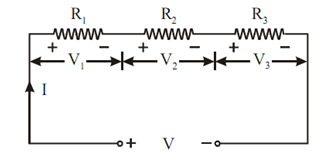## Voltage and Current Division in Resistive Circuits Assignment Help

Assignment Help: >> Resistance - Voltage and Current Division in Resistive Circuits

Voltage and Current Division in Resistive Circuits:

The corresponding resistance of series combination of resistances is the addition of all resistances connected in series.

Req = R1 + R2 + R3Figure: Resistances in Series

In series circuit, current remains similar through all of the resistances whereas the voltage is divided in all resistances.

V = V1 + V2 +V3

= IR1 + IR2 + IR3

= I (R1 + R2 + R3) = I Req

 Current Division Rule Voltage Division Rule#### Assured A++ Grade

Get guaranteed satisfaction & time on delivery in every assignment order you paid with us! We ensure premium quality solution document along with free turntin report!

All rights reserved! Copyrights ©2019-2020 ExpertsMind IT Educational Pvt Ltd